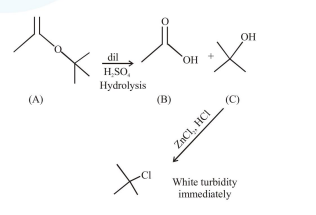# An organic compound (A) (molecular formula C6H12O2) was hydrolysed with dil.

Question:

An organic compound (A) (molecular formula $\mathrm{C}_{6} \mathrm{H}_{12} \mathrm{O}_{2}$ ) was hydrolysed with dil. $\mathrm{H}_{2} \mathrm{SO}_{4}$ to give a carboxylic acid (B) and an alcohol (C). ${ }^{\prime} \mathrm{C}^{\prime}$ give white turbidity immediately when treated with anhydrous $\mathrm{ZnCl}_{2}$ and conc. $\mathrm{HCl}$. The organic compound (A) is :

1.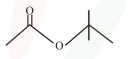2.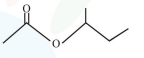3.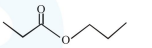4.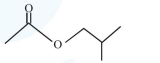Correct Option: 1

Solution: